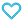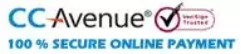# Rank Up Physics JEE Main & Advanced Mechanics Volume-2

350.00

• Paper Book

Discount offer on this book in a bundle, click to view

MTG’s Rank Up Physics for JEE Main & Advanced is a Physics book series comprising 5 books. The series is created by Physics experts to ensure that a JEE (M+A) aspirant can cover the Physics syllabus of JEE exam from all dimensions. The book is an excellent companion for students who wish to learn physics from scratch.
To develop a strong understanding of concepts, the chapters are well explained in a simple language and along with solved intext examples and fundamental questions. The exercises and previous years’ questions in each volume are well equipped with answer keys and explanations so that the student can self-evaluate their understanding of concepts.

147 in stock

Qty:Add to WishlistMTG’s Rank Up Physics is an exhaustive book series for the preparation of the Physics syllabus of JEE Main & Advance. The book series includes Five books namely-
• Mechanics Volume 1 (Covers: Basic Mathematics, Unit, Dimensions, and Errors, Vectors, Motion, Projectile Motion, Laws of Motion, Work, Energy and Power, Circular Motion, and Centre Of Mass and Conservation of Momentum)
• Mechanics Volume 2 (Covers: Rotational Motion, Gravitation, Elasticity, Fluid Mechanics, Simple Harmonics)
• Waves And Thermodynamics (Covers: Wave Motion, Superposition of waves, Sound Waves, Thermal Properties and Kinetic Theory, Thermodynamics and Heat Transfer)
• Electricity And Magnetism (Covers: Electrostatics, Capacitors, Electric Current, Magnetic Force, and Magnetic Field, Electromagnetic Induction, and Alternating Current)
• Optics And Modern physics (Covers: Geometrical Optics, Wave Optics, Atomic Physics, Nuclear Physics)
The uniqueness of this series lies in the following fact:
• Complete coverage of syllabus.
• Simplified presentation of complex Physics concepts.
• Numerous solved examples, questions & numerical for each chapter.
• Variety of questions like – single correct answer type, multiple correct answer type, passage-based, matrix match, integer type, etc. to prepare you for both JEE Main & Advanced.
• Previous 2 years’ (2020 & 2021) questions of JEE Main and JEE Advanced with detailed solutions.

Table of Content:
• Unit 1: Rotational Motion
• Introduction
• Kinematics of a Rigid Body
• Rotational Variables
• Torque
• Equilibrium
• Angular Momentum
• Toppling
• Moment of Inertia
• Rotational Kinetic Energy of a Rigid Body Rotating about a Given Axis
• Acceleration of a Point on a Fixed Rotating Object
• Rotation About a Fixed Axis
• Angular Impulse
• Rolling
• Instantaneous Axis of Rotation
• Dynamics of Rolling
• Rolling on a Inclined Plane
• Collision Involving Rotational Motion
• Exercise
• Unit 2: Gravitation
• Newton’s Law of Gravitation
• Characteristics of Gravitational Force
• Variation in Acceleration due to Gravity
• Gravitational Field
• Gravitational Potential
• Gravitational Field and Potential due to Continuous Bodies
• Gravitational Potential Energy
• Maximum Height of a Projectile
• Relation between Gravitational Field and Potential
• Kepler’s Laws
• Geostationary Satellite
• Double Star System
• Motion of Elliptical Orbits
• Analogy between Gravitation and Electrostatics
• Exercise
• Unit 3: Elasticity
• Elasticity
• Stress and Strain
• Hooke’s Law
• Stress-Strain Graph
• Types of Stress
• Potential Energy Stored in a Deformed Object
• Poisson’s Ratio
• Thermal Stress
• Exercise
• Unit 4: Fluid Mechanics
• Fluid Statics
• Density
• Pressure in a Fluid
• Pascal’s Law
• Variation of Pressure in Static Liquids
• Variation of Pressure in Accelerating Fluids
• Hydrostatic Forces on Submerged Surfaces
• Fluid Dynamics
• Fluid Flow Description
• Principle of Continuity
• Applications of Bernoulli’s Theorem
• Viscosity
• Newton’s Law of Viscous Flow
• Stokes’ Law
• Terminal Velocity
• Reynold’s Number
• Poiseuille’s Equation
• Surface Tension
• Explanation of Surface Tension
• Excess Pressure inside a Liquid Drop
• Angle of Contact
• Rise/Depression of Liquid in a Capillary Tube
• Rise of Liquid between Parallel Plates
• Exercise
• Unit 5: Simple Harmonic Motion
• Periodic Motion
• Simple Harmonic Motion
• Linear SHM
• Displacement, Velocity and Acceleration in SHM
• Phase
• Relation between SHM and Uniform Circular Motion
• Energy of a Particle in SHM
• Role of Potential Energy in SHM
• Time Period in SHM
• Time Period of SHM for Combination of Spring
• Time Period of Simple Pendulum
• SHM Involving Concept of Rotation
• SHM in Fluids
• SHM Involving Concepts of Heat and Thermodynamics: Oscillations of a Piston
• SHM Involving Concepts of Gravitation
• Physical Pendulum or Compound Pendulum
• Torsional Pendulum
• Two Body Oscillations
• Superposition of Two SHM’s
• Exercise